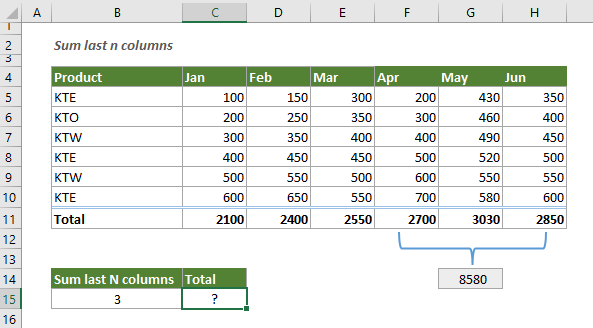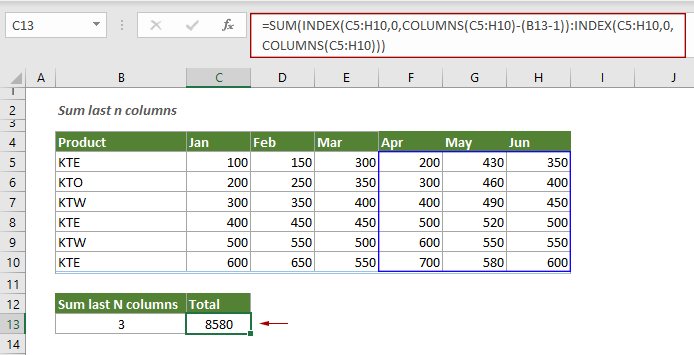## Sum the last N columns in Excel

To sum values in the last n columns, normally you may need to calculate the sum of each column and then add up the results to get the final sum. This tutorial provides a formula based on the INDEX function to help you easily get it done.

#### How to sum values in the last n columns in Excel?

As shown in the example below, there is a monthly sales table, to sum only the sales in the last 3 months (Apr, May and Jun), you can follow the steps below.Generic Formulas

=SUM(INDEX(data_range,0,COLUMNS(data_range)-(n-1)):INDEX(data_range,0,COLUMNS(data_range)))

Arguments

Data_range: A range of cells where we want to sum the last n columns;
N: A number represents the number of last columns you will sum from the data_range.

How to use this formula?

Select a formula, copy or enter the formula below and press the Enter key to get the result.

=SUM(INDEX(C5:H10,0,COLUMNS(C5:H10)-(B13-1)):INDEX(C5:H10,0,COLUMNS(C5:H10)))Note: In this case, cell B13 contains the number representing the last N columns, you can directly type this number into the formula and enclose it in double quotation marks as follows.

=SUM(INDEX(C5:H10,0,COLUMNS(C5:H10)-("3"-1)):INDEX(C5:H10,0,COLUMNS(C5:H10)))

Explanation of the formula

=SUM(INDEX(C5:H10,0,COLUMNS(C5:H10)-(B13-1)):INDEX(C5:H10,0,COLUMNS(C5:H10)))

INDEX(C5:H10,0,COLUMNS(C5:H10)-(B13-1)):
COLUMNS(C5:H10): The COLUMNS function returns the number of columns in the reference C5:E4. Here you will get 6 columns;
INDEX(C5:H10,0,6-(B13-1)) = INDEX(C5:H10,0,6-(3-1)) = INDEX(C5:H10,0,4): The syntaxt of the INDEX function: INDEX(array, row_num, [column_num]). Here the row_number is set to 0, so the INDEX function returns the reference for the entire fourth column: \$F\$5:\$F\$10;
INDEX(C5:H10,0,COLUMNS(C5:H10))
COLUMNS(C5:H11): The COLUMNS function returns the number of columns in the reference C5:E4. Here you will get 6 columns;
INDEX(C5:H10,0,6)): Here the INDEX function returns the reference for the entire sixth column: \$H\$5:\$H\$10;
SUM(\$F\$5:\$F\$10:\$H\$5:\$H\$10) = SUM(\$F\$5:\$H\$10): The SUM function then sums all numbers in range F5:H10 and returns the total amount: 8580.

#### Related functions

Excel SUM function
The Excel SUM function adds values.

Excel INDEX function
The Excel INDEX function returns the displayed value based on a given position from a range or an array.

Excel COLUMNS function
The Excel COLUMNS function returns the total number of columns in a given array or reference.

#### Related formulas

Sum multiple columns if one criterion is met
This tutorial will guide you how to sum values in multiple columns based on the same criterion in Excel.

Sum top N values or top N values with criteria
This step-by-step tutorial demonstrates how to use the SUMPRODUCT function with the LARGE function to sum the top N values in a range and sum the top N values in a range with criteria in Excel.

Sum values based on column or both column and row
This tutorial provides different formulas based on the SUMPRODUCT function to help you sum values based on a column header, and sum values based on both column and row criteria.

Sum values in last N days based on criteria
To sum values in last N days based on specific criteria in Excel, you can apply a formula based on the SUMIF and TODAY function.

### The Best Office Productivity Tools

#### Kutools for Excel - Helps You To Stand Out From Crowd

 Popular Features: Find/Highlight/Identify Duplicates  |  Delete Blank Rows  |  Combine Columns or Cells without Losing Data  |  Round without Formula ... Super VLookup: Multiple Criteria  |  Multiple Value   |  Across Multi-Sheets  |  Fuzzy Lookup... Adv. Drop-down List: Easy Drop Down List  |  Dependent Drop Down List  |  Multi-select Drop Down List... Column Manager: Add a Specific Number of Columns  |  Move Columns  |  Unhide Columns  |  Compare Columns to Select Same & Different Cells ... Featured Features: Grid Focus  |  Design View  |  Big Formula Bar  |  Workbook & Sheet Manager  |  Resource Library (Auto Text)  |  Date Picker  |  Combine Worksheets  |  Encrypt/Decrypt Cells  |  Send Emails by List  |  Super Filter  |  Special Filter (filter bold/italic/strikethrough...) ... Top 15 Toolsets:  12 Text Tools (Add Text, Remove Characters ...)  |  50+ Chart Types (Gantt Chart ...)  |  40+ Practical Formulas (Calculate age based on birthday ...)  |  19 Insertion Tools (Insert QR Code, Insert Picture from Path ...)  |  12 Conversion Tools (Numbers to Words, Currency Conversion ...)  |  7 Merge & Split Tools (Advanced Combine Rows, Split Cells ...)  |  ... and more

Kutools for Excel Boasts Over 300 Features, Ensuring That What You Need is Just A Click Away...

Supports Office/Excel 2007-2021 and 365   |   Available in 44 Languages   |   Easy to Uninstall Completely#### Office Tab - Enable Tabbed Reading and Editing in Microsoft Office (include Excel)

• One second to switch between dozens of open documents!
• Reduce hundreds of mouse clicks for you every day, say goodbye to mouse hand.
• Increases your productivity by 50% when viewing and editing multiple documents.
• Brings Efficient Tabs to Office (include Excel), Just Like Chrome, Edge and Firefox.